Genetic algorithm for project time-cost optimization in fuzzy environment

Genetic algorithm for project time-cost optimization in fuzzy environment

Khan Md. Ariful Haque, Md. Ahsan Akhtar Hasin

Rajshahi University of Engineering & Technology (Bangladesh)

Accepted: August 2012

Ariful Haque, K.M., & Hasin, M.A.A. (2012). Genetic algorithm for project time-cost optimization in fuzzy environment. Journal of Industrial Engineering and Management, 5(2), 364-381. http://dx.doi.org/10.3926/jiem.410

---------------------

Abstract:

Purpose: The aim of this research is to develop a more realistic approach to solve project time-cost optimization problem under uncertain conditions, with fuzzy time periods.

Design/methodology/approach: Deterministic models for time-cost optimization are never efficient considering various uncertainty factors. To make such problems realistic, triangular fuzzy numbers and the concept of a-cut method in fuzzy logic theory are employed to model the problem. Because of NP-hard nature of the project scheduling problem, Genetic Algorithm (GA) has been used as a searching tool. Finally, Dev-C++ 4.9.9.2 has been used to code this solver.

Findings: The solution has been performed under different combinations of GA parameters and after result analysis best values of those parameters have been found for the near optimal or sustainable solution.

Research limitations/implications: For demonstration of the application of the developed algorithm, a project on new product (Pre-paid electric meter, a project under government finance) launching has been chosen as a real case. The formulation of the model of the problem is developed under some assumptions that have been mentioned in section 5.

Practical implications: The proposed model leads decision makers to choose the desired solution under different risk levels.

Originality/value: Reports reveal that project optimization problems have never been solved under multiple uncertainty conditions. Here, the function has been optimized using Genetic Algorithm search technique, with varied level of risks and fuzzy time periods.

Keywords: time-cost optimization, fuzzy time functions, risk levels, genetic algorithm

---------------------

1. Introduction

A project is a combination of interrelated activities which must be executed in a certain order before the entire task is completed. The activities are interrelated in a logical sequence which is known as precedence relationship. For the analytical purpose, the activities of a project are represented in a network diagram maintaining precedence relationship to get solutions for scheduling and controlling. The longest continuous path of a project network is called critical path which determines the project duration. The most commonly used project management techniques are Gantt chart, Milestone, Critical Path Method (CPM) and Project Evaluation and Review Technique (PERT). The major objective of project scheduling is to complete the entire project within budget and time constraints. Traditional project scheduling problems mainly focus on activities assuming deterministic or probabilistic time durations.

Project scheduling is the conversion of a project action plan in an operating timetable. It serves as the basis for monitoring and controlling project activity. Taken together with the plan and budget, it is probably the major tool for the management of projects. The basic approach for all scheduling techniques is to form a network of activity and event relationships. Critical path of this network is an important issue for project schedulers, because it refers the duration of whole project. Project managers are highly concerned with this critical path for on time completion of a project, especially when an extension will incur a penalty (either in liquidated damages, opportunity costs and goodwill losses). When some delay have been occurred, there may be necessary to compress the critical activity by incrementing the activity resources above the normal level. It is often the case that the performance of some or all activities can be accelerated, or the duration crashed, by allocating more resources at the expense of higher activity direct cost. This crashing of activities can be achieved by multiple shift work, extended work days, using larger and more productive equipment and increasing the size of labour crews. So, project schedule planners mainly focus on finding the most cost effective way to complete a project within a specified completion time. This class of problem is usually called time-cost trade off.

In real construction projects, time and cost of activities may face significant changes due to existing uncertainties such as inflation, economical and social stresses, labour performance, execution errors of contractor, design errors, natural events such as climate changes and etc. Therefore, total time and cost of project may differ significantly because of these uncertainties.

Almost all of the projects, the required information for estimation of project parameters are either unavailable or incomplete. In practice, the majority of construction companies do not systematically record the durations of project activities. In addition, it is commonly known that no two construction projects are alike. Also in many cases the project is done for the first time, this compels us to use expert opinion in forecasting the project parameters. Experts use their own judgment, experience and project information that is available to them. In practice, linguistic terms such as, “approximately”, “more or less”, or “about” are commonly found in the statements used by these experts. These terms clearly exhibit some sort of imprecision that naturally leads to a range of possible values, rather than a definitive estimate using a single value. So, uncertainty and project parameters are inextricable. In thesis situation, deterministic models of construction time-cost trade off are not realistic. Crisp decision making in uncertain environment causes loss of some parts of information. Use of uncertain models, whish is capable of formulating vagueness of the dynamic conditions of real world gives more stability to solve time-cost trade off problem. Some probabilistic techniques are used in many cases to meet these uncertainties to some extent. But project parameters may not be in statistical manner. That is why fuzzy set theory is appropriate to consider affecting uncertainties in activity duration, direct and indirect cost of a project.

Since different alternatives of possible durations and costs for the activities can be associated with a project, the problem is arisen to search the best solution. As combinatorial optimization problems, finding optimal decisions is difficult and time consuming considering the number of possible permutations involved. Therefore, any analytical procedure may suit for small project, but it will be inefficient for large project because of exhaustive enumeration. To avoid the problem of combinatorial explosion, heuristic models can be used. It does not ensure the optimality but it gives better near optimal solution without mathematical rigor.

This research aimed at development of an efficient heuristic approach with fuzzy activity time and cost for project time-cost optimization incorporating the vagueness or fuzziness of the dynamic conditions of the real world.

2. Literature review

Scheduling of project activities with minimum cost is one of the concerned fields of project management to avoid the penalties incurred for delaying the project completion time. Hence, it is becoming as one of the most fundamental and essential bases of research interest of many researchers.

Considering the importance of construction time-cost optimization, various analytical and heuristic methods have been proposed by many researchers in recent decades.

Some examples of heuristic approaches are Fondahl’s (1961) method, Prager’s (1963) structural model, Siemens’s (1971) effective cost slope model, and Moselhi’s (1993) structural stiffness method. Siemens has developed SAM (Siemens Approximation Method) algorithm which is ideally suited for hand computation and also is suitable for computer solution. Lakshminarayanan, Gaurav and Arun (2011) developed a meta-heuristic multi-colony ant algorithm for optimization of three objectives time-cost-risk as trade-off problem.

Feng, Liu and Burns (1997) developed a GA model that is an improvement of their earlier linear programming / integer programming model (Liu, Burns & Feng, 1995). The model by Li and Love (1997), on the other hand, was formulated to produce the times, in real numbers, by which each critical activity should be reduced. The study did not consider the formation of other critical paths during the crashing process and was limited to continuous, as opposed to discrete, variables for crashing times. Hegazy (1999) developed a practical GA model by implementing the GA protocols within Microsoft Project. This model has demonstrated an improvement over the previous GA models. Zheng, Ng and Kumaraswamy (2004) has introduced a multi-objective model by using genetic algorithms integrates the adaptive weight derived from previous generations and induces a search pressure toward an ideal point. Chua, Chan and Govinda (1997) proposed a time-cost trade-off model using genetic algorithm. Li, Hussein and Lei (2011) proposed a methodological framework including optimization, sensitivity analysis, and improved GA for build-operate-transfer projects. However, the above mentioned time-cost trade-off models mainly focus on deterministic environment.

Uncertainties (such as inflation, economical and social stresses, execution errors of contractor, design errors, natural events as climate changes, etc.) in the problem have received less attention due to its complexity. These uncertainties cause changes in durations and cost of activities and hence total cost of project may differ significantly. Because of its practical relevance researchers have recently begun to pay special attention to uncertain scheduling.

Han, Chung and Liang (2006) has used fuzzy critical path method to improve fuzzy airport’s ground operation decision analysis assuming fuzzy activity times as trapezoidal fuzzy number. They also utilized a fuzzy ranking method developed by Ling and Han (2004) in fuzzy CPM. Chanas and Zielinski (2001) presented two of calculation of the path degree of criticality with application of the extension principle of Zadeh. Liu (2003) formulated the critical path and the project crashing problems by linear programming with fuzzy activity times and then defuzzify the fuzzy activity times following Yager’s (1981) ranking method. Lorterapong and Moselhi (1996) presented an approach of project-network analysis based on fuzzy sets theory. His approach overcomes the limitations of backward pass calculations to compute project completion time with uncertainty. To solve time-cost trade-off problem, Ghazanfari (2008) developed an approach by possibility goal programming with fuzzy decision variables. It could not fully satisfy the real field problem of time-cost trade-off problem as crashing costs are crisp.

Leu, Chen and Yang (2001) incorporated fuzzy set theory with genetic algorithms to model uncertainty in time-cost trade-off problem. Project scheduling with resource consideration was also developed by Leu, Chen and Yang (1999). Abbasnia, Afshar and Eshtehardian (2008) have investigated fuzzy logic based approach called Non-dominated Sorting Genetic Algorithm (NSGA) for time-cost trade-off problem in uncertain environment. This model cannot fully meet uncertainty of practical problem.

Other than fuzzy time-cost trade-off problem, a probabilistic model has been developed by Arazon, Perkgoz and Sakawa (2005) in PERT network using a genetic algorithm. Another analytical method that combines fuzzy set theory with the PERT technique for measuring the criticality in a project network was developed by Chen and Huang (2007).

3. Fuzzy set theory

Concept of fuzzy numbers

There are different forms of fuzzy numbers. This paper only considers triangular fuzzy numbers that can be defined by a triplet Â=(a,b,c) the membership function is;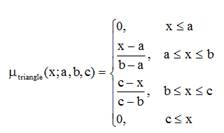The parameter b is the centre point, a and c are two base points as in Figure 1. It shows that degree of membership of a, b and c are 0, 1 and 0 respectively.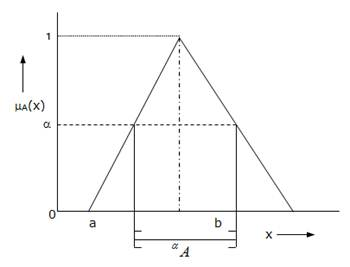Figure 1. Triangular fuzzy membership function and a-cut level

Concept of alpha (a)-cut

The a-cut is a commonly used method to connect the principles of fuzzy sets with a collection of crisp sets, which can in turn be fed into most of the existing systems. Given a fuzzy set A defined on X and any number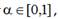, the a-cut, aA is the crisp set as defined by: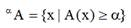That is, the a-cut of a fuzzy set A is the crisp set aA that contains all the elements of the universal set X whose membership grades in A are greater than or equal to the specified value of a which is clearly explained by Figure 1.

In this paper, a-cut is known to incorporate the experts or decision makers’ confidence over his preference or the judgments. a =1 means the expert is highly certain about his knowledge regarding a phenomenon over which he expresses his preferences then the outcome will be a single value having the membership 1 in the fuzzy performance set. When a <1, it indicates there exist uncertainty. a =0 expresses the highest level of uncertainty. Actually a represents the degree of risk that the managers is prepared to face.

4. Genetic algorithm (GA)

Genetic algorithm is a heuristic search approach that provides a means of optimization of NP-hard problems. These procedures combine an artificial survival-of-the-fittest strategy with genetic operators abstracted from nature (Goldberg, 1989), to form a mechanism that is suitable for a variety of optimization problems. The theory behind GA is that a population of certain species will, after many generations of random evolutions, adapt to live better in its environment. GA solves optimization problems in the same fashion. This procedure begins by generating an initial population of random solutions that are codified in the strings of numbers called chromosomes. Each individual chromosome represents one solution that is better, or worse, than others in the population. The fitness of each solution is determined by evaluating its performance with respect to an objective function named as fitness function. A selection mechanism is applied to the current population to create an intermediate population. Then crossover and mutation operators are applied to the intermediate population to form a novel population. This population is then used in the next iteration of the algorithm. Usually, this process is continued for a large number of offspring generations in which the population keeps evolving (better solutions replace unfit solutions), until a terminating criterion is met. At the end of the process, the member of the population with the best performance becomes the optimum solution. The operations of GA process are shown in Figure 2.Figure 2. The GA process

5. Problem formulation

The objective of this model is to find the optimal combination of activity durations with minimum total project cost at different risk levels (a-cut levels) so that the project can be completed within a specified project duration selected from feasible project time spectrum. The mathematical model is expressed as follows:Where,

aC= Total project cost at a specific a-cut level

adi= Duration of activity i at a specific a-cut level

aCNi= Normal cost of activity i at the duration adi

aCCi= Crash cost of activity i at the duration adi

aCJi= Indirect cost of activity i at the duration adi

aNi= Normal cost rate of activity i at the duration adi

aNCi= Crash cost rate of activity i at the duration adi

aNJi= Indirect cost rate of activity i at the duration adi

aT= Project duration at a specific a-cut level

D = Permissible maximum project duration

adimin= Minimum duration of activity i at a specific a-cut level finished in crash

adilow= Minimum duration of activity i at a specific a-cut level finished normally

adimax= Maximum duration of activity i at a specific a-cut level finished normally

Equation (1) indicates the total project cost. Equation (2) restricts the project must be finished within the targeted duration. Equation (3) ensures each activity time is restricted between minimum crash duration and maximum normal duration. This model is formulated on the basis of some assumptions. These are all activities are crashable, normal and crash activity durations are characterized by triangular fuzzy numbers, direct and indirect cost rates are also characterized by triangular fuzzy numbers, durations of activities are considered only integer values within its range, direct cost rates are decreased and indirect cost rates are increased as activity duration increases in fuzzy nature, and resources are not limited.

6. Fuzzy relationship between time and cost

Figure 3 depicts the concept of activity durations and their corresponding costs generation procedure. The activity duration can be divided into three regions- crash time (region-1), normal time (region-2) and overlapping time (region-3) as in Figure 3(a). Within the crash time, the activity needs to be performed in a crash mode, i.e., it needs to spend great deal of effort to reduce the time. Within the normal time, the activity can be finished in normal mode. The cost in a crash mode is generally higher than that in a normal mode since more resources are needed to invest in the activity so as to finish as early as possible. When the activity time falls within the overlapping region, it can be performed in either normal mode or crash mode. But it is reasonable to finish the activity in normal mode as it will be cost effective.

At a specific a-cut level, points h, g, f, e in Figure 3 (b) represent corresponding direct cost rates of points a, c, b and d in Figure 3(a) respectively. In case of indirect cost, points i and k in Figure 3(c) represent corresponding indirect cost rates of points a and d in Figure 3(a) respectively. Direct and indirect cost rates of other durations in the acceptable region are then calculated. These cost rates are used to determine the total costs (direct cost + indirect cost) of each activity which are the inputs of the next steps.

With the objective function, total project duration is determined by the critical path and the total cost of the project can be calculated at a specific a-cut level. To test the feasibility of the solution, project duration is compared with targeted completion time. The fittest solution would be the solution which has minimum total cost.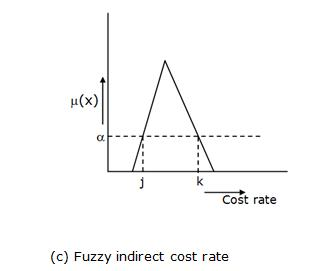Figure 3. Fuzzy relationships between time and cost of an activity

7. GA based solver

The operational architecture of GA solver for fuzzy time-cost optimization of a project is shown in Figure 5. GA solver starts with the creation of an initial population pool where each solution is presented as a string (chromosome) of activity durations. The initial population number is taken as computer input for the program. In Figure 4, chromosome 1 (parent) and chromosome 2 (parent) show sample encoded chromosomes that have been used in this paper. Numbers in cells represent activity durations of seven activities from A to G successively. A value of a-cut level and specified project duration (within feasible region) are also taken as input. After evaluating the fitness function, probability pi and cumulative probability qi for i chromosomes (where i = 1, 2, 3, ….) have been calculated. Roulette wheel mechanism is used to select chromosomes which will undergo breeding to create next generation.

In order to exploit and explore potential solutions, genetic algorithm generates possible chromosomes by using crossover and mutation operators. Single-point crossover is used in this solution. A crossover rate pc is taken indicates how many chromosomes will undergo the crossover operation. Figure 4 shows single-point crossover operation. Here, offspring 1 and offspring 2 have been created from their parent chromosomes by exchanging all bits after randomly chosen crossover point (crossover point is 3).

 Chromosome 1 26 12 16 7 6 4 5 Chromosome 2 29 14 20 3 3 5 6 Offspring 1 26 12 16 3 3 5 6 Offspring 2 29 14 20 7 6 4 5

Figure 4. Single point crossover operation

A mutation rate pm is used to control the percentage of bits on which mutation is applied. The bits in chromosomes are selected at random which will undergo the mutation operation. The selected bits will be swapped with the randomly selected activity duration within its acceptable region at the specified a-cut level. A generation is completed and a new set of population (offspring) has been created. Evaluate the fitness function value for the new population and save the best value. This process of reproduction will continue until predefined number of generations (stopping condition) is met. The overall GA problem solving approach is represented in Figure 5.Figure 5. GA problem solving approach

8. A case study and result analysis

The project on launching of a new product (pre-paid electric meter, a project under the government finance) is presented with a 7-activity CPM network (AOA diagram) illustrating project scheduling with time-cost trade-off problem. The precedence relationships of the network are depicted in Figure 6. The durations, direct and indirect cost rates for both normal and crash modes of each activity are shown in Table 1. The optimistic and pessimistic project duration margins with different values of a-cut levels are defined in Table 2 and Figure 7. Only integer activity times have been considered to estimate project duration. The region bounded by the optimistic and pessimistic margins is the possible project duration spectrum for construction time-cost trade-off.Figure 6. CPM network of the project on new product (Pre-paid electric meter, a project under government finance) launching

 Activity No. Name of the Activity Duration (day) Direct cost rate (Tk./day) Indirect cost rate (Tk./day) Crash Normal Crash Normal A Forecast sales volume, using market research 15,17,20 25,27,30 300,400,445 150,200,250 70,70,70 B Design pre-paid meter 10,12,15 13,16,20 200,250,260 100,120,130 20,20,20 C Materials and component procurement 15,16,20 16,20,26 200,250,300 80,120,170 60,60,60 D Laboratory test of materials and component 2,4,5 5,7,8 150,220,280 50,90,120 80,80,80 E Proto-type Production 2,3,5 4,6,7 290,370,410 100,160,210 100,100,100 F Laboratory and commercial testing 2,3,4 3,5,7 140,225,350 50,90,130 50,50,50 G Commercial production, sales and distribution planning 3,5,6 4,6,9 150,210,250 40,95,140 30,30,30

Table 1. Durations and cost rates of activities of the project on new product Pre-paid electric meter, under government finance) launching

 a Crash duration (day) Normal duration (day) Min Max Min Max 0.0 35 51 49 72 0.1 39 47 53 68 0.2 39 47 53 67 0.3 39 46 54 66 0.4 39 45 54 64 0.5 39 45 54 64 0.6 41 43 58 63 0.7 41 42 58 60 0.8 41 41 59 60 0.9 41 41 59 59 1.0 41 41 59 59

Table 2. Optimistic and pessimistic project duration margins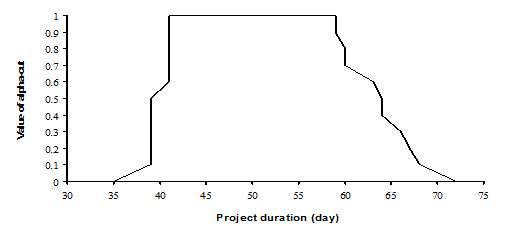Figure 7. Possible project duration spectrums of the project

The proposed GA based fuzzy time-cost optimization solver was coded in Dev-C++ 4.9.9.2 and run on a personal computer having Intel(R) Pentium(R) Dual CPU E2200 @ 2.20GHz and 2GB of RAM. Two folders were created: one is input folder and the other is output folder. All inputs are given into the input folder. GA operational parameters are given as input through dialogs and the all output data are exported to output folders for data analysis. Table 3 shows the results produced with the parameters set as number of initial population = 100, generation number = 10,000, project completion time £ 55 days. Here, a-cut value was varied. The best result was found at crossover rate = 0.8, and mutation rate = 0.1. For a-cut value=2, the result reveal that under time constraint of 55 days, the project manager should try to complete this project within 55 days with cost of Tk.16384.3 considering 0.2 (lower) risk levels.

 Values of a-cut level Durations (day) of activities Optimum project duration (day) Optimum Cost (Tk.) Computation time (second) A B C D E F G 0.0 30 13 19 2 2 3 4 55 15167.5 23.37 0.2 29 14 18 3 3 4 5 55 16384.3 23.30 0.4 28 15 19 3 3 4 5 55 16678.2 22.20 0.6 27 15 19 7 3 5 6 55 17147.4 20.01 0.8 27 16 20 7 3 5 5 55 17630.0 20.01 1.0 27 16 20 7 3 5 5 55 17630.0 19.00

Table 3. Effect of a-cut value on the optimum solution

An analysis found that as the alpha-cut value increases, the total cost of a project also increases (see Figure 8). It is because, the choice become limited for the selection of smaller value from possible duration of each activity to reduce the total cost of the project. Alternatively, it can be said that total cost of a project increases with the risk level (a-cut value) increases.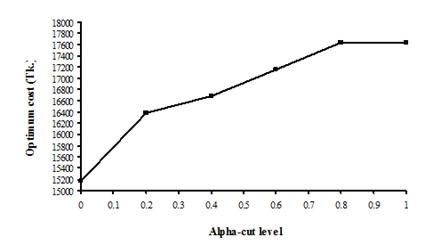Figure 8. Impact of alpha-cut level on project total cost

9. Convergence analysis

Figure 9 shows the convergence graph of the problem solving with the GA based solver. A convergence analysis is performed using the best values in each generation. This figure is drawn from the data with parameters of 0.2 a-cut level, project completion time £ 55 days, 100 number of initial population, Pc = 0.8 and Pm = 0.1. It is observed from the figure that the optimum value is improving with generation number and it is converging toward global optimum. After 4960 generations the global optimum solution has been found and its value is Tk.16384.3 only.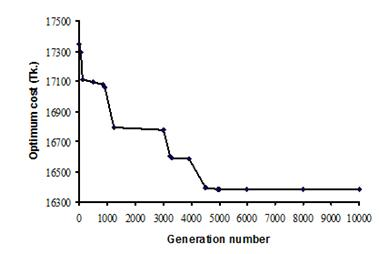Figure 9. Convergence graph of the project

10. Conclusions

This research work customized GA based project time-cost optimization algorithm in a fuzzy environment. It provides an efficient computational technique for time-cost optimization project scheduling problem incorporating uncertainty in network analysis. Because of dynamic situation of environment, these proposed algorithms are efficient due to fuzziness of its variables. With the concept of alpha-cut method of fuzzy theory, fuzzy input variables were transformed into crisp values. Due to NP-hard nature of the problem, a computer code of GA based solver was used to find the optimum solution within project completion time constraint at different values of a-cut level. Decision makers (mainly project managers) can use this model to choose the desired (optimum) solution for time-cost trade off within a time limit under different risk levels that varies with the values of a (a =0 means lowest level of risk and a =1 means highest level of risk).

The problem was resolved under different combinations of GA parameters. After analysis of results, optimum values of those parameters were found.

The performance of the presented GA based algorithm can be further analyzed in terms of CPU time by comparing it with other best known algorithms for project time-cost optimization.

Different selection mechanisms can change the algorithm efficiency. In this problem Roulette wheel selection method is suggested. A new selection method can be created as an attempt to improve the solution quality.

Crossover and mutation operators are important parameters of genetic algorithm. In this paper, single point crossover and random mutation operations were used in every generation. Other new types of crossover and mutation operations can be developed which may be respondent to the generation quality.

The algorithm can also be extended by considering resource limited time-cost optimization with other types of fuzzy numbers.

References

Abbasnia, R., Afshar, A., & Eshtehardian, E. (2008). Time-cost trade-off problem in construction project management, based on fuzzy logic. Journal of Applied Sciences, 8(22), 4159-4165. http://dx.doi.org/10.3923/jas.2008.4159.4165

Arazon, A., Perkgoz, C., & Sakawa, M. (2005). A genetic algorithm approach for the time-cost trade-off in PERT networks. Applied Mathematics and Computation, 168, 1317-1339. http://dx.doi.org/10.1016/j.amc.2004.10.021

Chanas, S., & Zielinski, P. (2001). Critical path analysis in the network with fuzzy activity times. Fuzzy Sets and Systems, 122, 195-204. http://dx.doi.org/10.1016/S0165-0114(00)00076-2

Chen, C. T., & Huang, S. F. (2007). Applying fuzzy method for measuring criticality in project network. Information Sciences, 177, 2448-2458. http://dx.doi.org/10.1016/j.ins.2007.01.035

Chua, D. K. H., Chan, W. T., & Govinda, K. (1997). A time-cost trade-off model with resource consideration using genetic algorithm. Civil. Eng. Syst., 14, 291-311. http://dx.doi.org/10.1080/02630259708970224

Feng, C., Liu, L., & Burns, S. (1997). Using genetic algorithms to solve construction time-cost trade-off problems. ASCE Journal of Computing in Civil Engineering, 11(3), 184-189. http://dx.doi.org/10.1061/(ASCE)0887-3801(1997)11:3(184)

Fondahl, J. W. (1961). A non-computer approach to the critical path method for the construction industry. Tech. Rep. No. 9, The Constr. Inst., Dept. of Civ. Engrg., Stanford Univ., Stanford, Calif.

Ghazanfari, M., Yousefli, A., Ameli, M. S. J., & Amiri, A. B. (2008). A new approach to solve time-cost trade-off problem with fuzzy decision variables. Int. J. Adv. Manuf. Technol, Doi. 10.1007/s00170-008-1596-y.

Han, T. C., Chung, C. C., & Liang, G. S. (2006). Application of fuzzy critical path method to airport’s cargo ground operation system. Journal of Marine Science and Technology, 14(3), 139-146.

Hegazy, T. (1999). Optimization of construction time-cost trade-off analysis using genetic algorithms. Canadian Journal of Civil Engineering, 26(6), 685-697. http://dx.doi.org/10.1139/l99-031

Klir, G. J., & Yuan, B. (2005). Fuzzy sets and Fuzzy logic: Theory and Applications. Prentice -Hall of India.

Lakshminarayanan, S., Gaurav, A., & Arun, C. (2011). Time-cost-risk trade off using ant colony optimization. Journal of Construction in Developing Countries, Preview Manuscript.

Leu, S. S., Chen, A. T., & Yang, C. H. (1999). Fuzzy optimal model for resource-constrained construction scheduling. Journal of Computing in Civil Engineering, 13(3), 207-216. http://dx.doi.org/10.1061/(ASCE)0887-3801(1999)13:3(207)

Leu, S. S., Chen, A. T., & Yang, C. H. (2001). A GA-based fuzzy optimal model for construction time-cost trade-off. International Journal of Project Management, 19, 47-58. http://dx.doi.org/10.1016/S0263-7863(99)00035-6

Li, H., Hussein, M. A., & Lei, Z. (2011). Incentive genetic algorithm based time–cost trade-off analysis across a build–operate–transfer project concession period. Can. J. Civ. Engrg., 38, 166-174. http://dx.doi.org/10.1139/L10-118

Li, H., & Love, P. (1997). Using improved genetic algorithms to facilitate time-cost optimization. ASCE Journal of Construction Engineering and Management, 123(3), 233-237. http://dx.doi.org/10.1061/(ASCE)0733-9364(1997)123:3(233)

Liang, G. S., & Han, T. C. (2004). Critical path analysis based on fuzzy concept. International Journal of Information and Management Sciences, 15(4), 29-40.

Liu, L., Burns, S., & Feng, C. (1995). Construction time-cost trade-off analysis using LP/IP. ASCE Journal of Construction Engineering and Management, 121(4), 446-454. http://dx.doi.org/10.1061/(ASCE)0733-9364(1995)121:4(446)

Liu, S. T. (2003). Fuzzy activity times in critical path and project crashing problems. Cybernetics and Systems, 34, 161-172. http://dx.doi.org/10.1080/01969720302865

Lorterapong, P., & Moselhi, O. (1996). Project-network analysis using fuzzy sets theory. Journal of Construction Engineering and Management, 122(4), 308-318. http://dx.doi.org/10.1061/(ASCE)0733-9364(1996)122:4(308)

Moselhi, O. (1993). Schedule compression using the direct stiffness method. Can. J. Civ. Engrg., 20, 65-72. http://dx.doi.org/10.1139/l93-007

Prager, W. (1963). A structural method of computing project cost polygons. Mgmt. Sci., 9(3), 394-404. http://dx.doi.org/10.1287/mnsc.9.3.394

Siemens, N. (1971). A simple CPM time-cost tradeoff algorithm. Mgmt. Sci., 17(6), B-354-363. http://dx.doi.org/10.1287/mnsc.17.6.B354

Yager, R. R. (1981). A procedure for ordering fuzzy subsets of the unit interval. Information Science, 24, 143-161. http://dx.doi.org/10.1016/0020-0255(81)90017-7

Zheng, D. X. M., Ng, S. T., & Kumaraswamy, M. M. (2004). Applying a genetic algorithm-based multiobjective approach for time-cost optimization. Journal of Construction Engineering and Management, 130(2), 168-176. http://dx.doi.org/10.1061/(ASCE)0733-9364(2004)130:2(168)